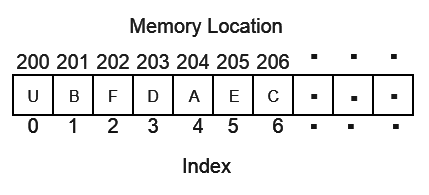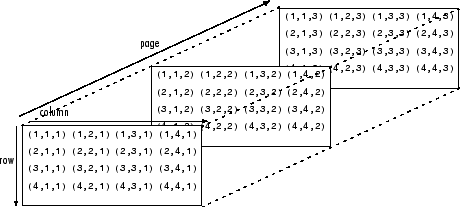# I. Write a detail note on Representation of single and multidimensional arrays.

Array is a data structure that is used to store variables that are of similar data types at contiguous locations. The main advantage of array is random access and cache friendliness. There are mainly 2 types of array.

Single Dimensional and Multidimensional.

Single Dimensional Array.

• It is a list of the variable of similar  data types.
• It allows random access and all the elements can be accessed with the help of their index.
• The size of the array is fixed.
• For a dynamically sized array, vector can be used in C++.
• Representation of 1D array:Multi Dimensional Array:

A multi-dimensional array can be termed as an array of arrays that stores homogeneous data in tabular form. Data in multidimensional arrays is generally stored in row-major order in the memory.

The general form of declaring N-dimensional arrays is shown below.

Syntax:

data_type array_name[size1][size2]….[sizeN];

• data_type: Type of data to be stored in the array.
• array_name: Name of the array.
• size1, size2,…, sizeN: Size of each dimension.

Examples:

Two dimensional array:

int two_d;

Three dimensional array:

int three_d;

### Size of Multidimensional Arrays:

The total number of elements that can be stored in a multidimensional array can be calculated by multiplying the size of all the dimensions.
For example:

• The array int x can store total (10*20) = 200 elements.
• Similarly array int x can store total (5*10*20) = 1000 elements.

Example: